GFG App
Open AppBrowser
Continue

# LINQ Operators and Methods for Transforming Data

LINQ (Language Integrated Query) is a set of language and rich library features provided by C# for writing consistent and intuitive syntax for querying data from various data sources, such as arrays, lists, collections, dictionaries, databases, and more in a type-safe and expressive way.

Some of the important functions of LINQ are:

• Filtering
• Sorting
• Transforming
• Grouping
• Joining
• Aggregation

In this article, we shall have detailed insights into the set of methods or query operators that can be used to transform the elements of a LINQ query from one type to another. These methods allow us to perform operations such as projection, restriction, partitioning, and conversion on the data in our queries.

Following are some of the methods that are used in transforming the data using LINQ:

## Projection Query Operators

### Select:

This method is used to transform each element of the query elements into the output sequence that contains the same number of transformed elements, based on a projection function that we specify.

Example 1:

## C#

 `using` `System; ` `using` `System.Linq; ` ` `  `public` `class` `GFG{ ` ` `  `    ``static` `public` `void` `Main (){ ` `        ``// Define a list of integers ` `        ``var` `numbers = ``new` `List<``int``> { 1, 2, 3, 4, 5 }; ` ` `  `        ``// Use the Select method to project the elements of the list ` `        ``// into a new form, by applying a transformation to each element ` `        ``var` `squaredNumbers = numbers.Select(x => x * x); ` ` `  `        ``// Output the elements of the transformed query ` `        ``foreach` `(``var` `num ``in` `squaredNumbers) ` `        ``{ ` `            ``Console.WriteLine(num); ` `        ``} ` `    ``} ` `}`

Output: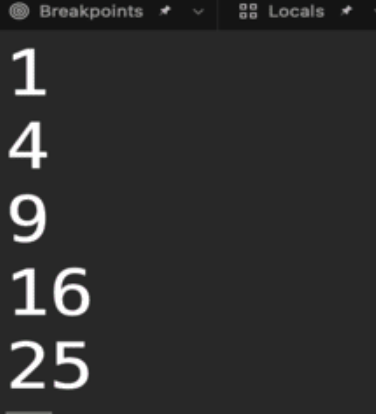### SelectMany:

This method is used to flatten the elements of a LINQ query that contains sequences or collections of elements. The SelectMany method applies to each element in the query and flattens into a single sequence.

Example 2:

## C#

 `using` `System; ` `using` `System.Linq; ` ` `  `public` `class` `GFG{ ` ` `  `    ``static` `public` `void` `Main (){ ` ` `  `        ``// Define a list of lists of integers ` `        ``var` `numbers = ``new` `List> ` `        ``{ ` `            ``new` `List<``int``> { 1, 2, 3 }, ` `            ``new` `List<``int``> { 4, 5, 6 }, ` `            ``new` `List<``int``> { 7, 8, 9 }, ` `        ``}; ` ` `  `        ``// Use the SelectMany method to flatten the lists of integers ` `        ``// into a single sequence of integers ` `        ``var` `flattenedNumbers = numbers.SelectMany(x => x); ` ` `  `        ``// Output the elements of the flattened query ` `        ``foreach` `(``var` `num ``in` `flattenedNumbers) ` `        ``{ ` `            ``Console.WriteLine(num); ` `        ``} ` `    ``} ` `}`

Output: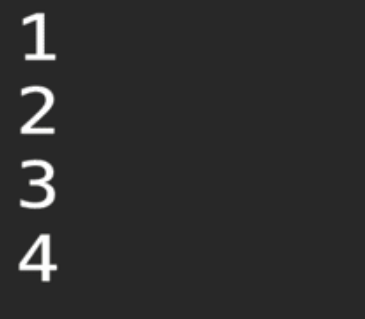## Restriction Query Operator

### Where:

This method is used to filter(“restrict”) and return the elements of a LINQ query based on a condition that we specify. The output of Where method contains only the elements that match a given predicate. The elements themselves individual are not modified or transformed.

Example 3:

## C#

 `using` `System; ` `using` `System.Linq; ` ` `  `public` `class` `GFG{ ` ` `  `    ``static` `public` `void` `Main (){ ` `       `  `        ``// Define a list of integers ` `        ``var` `numbers = ``new` `List<``int``>{1, 2, 3, 4, 5, 6, 7, 8, 9}; ` ` `  `        ``// Use the Where method to filter the elements of the list ` `        ``// based on a condition that you specify ` `        ``var` `evenNumbers = numbers.Where(x => x % 2 == 0); ` ` `  `        ``// Output the elements of the filtered query ` `        ``foreach` `(``var` `num ``in` `evenNumbers) ` `        ``{ ` `            ``Console.WriteLine(num); ` `        ``} ` `    ``} ` `}`

Output: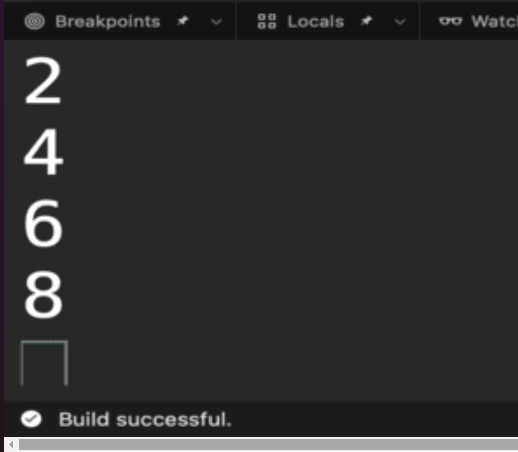## Partitioning Query Operators

These methods are used to return a specified number of elements from the start of a LINQ query, or while a condition is true.

• Take: This method returns the output sequence with specified number of elements from the start of the query.
• TakeWhile: This method returns elements from the start of the query when a specified condition is matched.

Example 4:

## C#

 `using` `System; ` `using` `System.Linq; ` ` `  `public` `class` `GFG{ ` ` `  `    ``static` `public` `void` `Main (){ ` `       `  `        ``// Define a list of integers ` `        ``var` `numbers = ``new` `List<``int``>{1, 2, 3, 4, 5, 6, 7, 8, 9}; ` `        ``// Use the Take method to return the first 3 elements of the list ` `        ``var` `firstThreeNumbers = numbers.Take(3); ` `       `  `        ``Console.WriteLine(``"Take method:"``); ` ` `  `        ``// Output the elements of the taken query ` `        ``foreach` `(``var` `num ``in` `firstThreeNumbers) ` `        ``{ ` `            ``Console.WriteLine(num); ` `        ``} ` `       `  `        ``// Use the TakeWhile method to return the elements of the list ` `        ``// while the elements are less than or equal to 3 ` `        ``var` `numbersLessThanOrEqualToThree = numbers.TakeWhile(x => x <= 3); ` `       `  `        ``Console.WriteLine(``"TakeWhile method:"``); ` ` `  `        ``// Output the elements of the taken query ` `        ``foreach` `(``var` `num ``in` `numbersLessThanOrEqualToThree) ` `        ``{ ` `            ``Console.WriteLine(num); ` `        ``} ` `    ``} ` `} `

Output: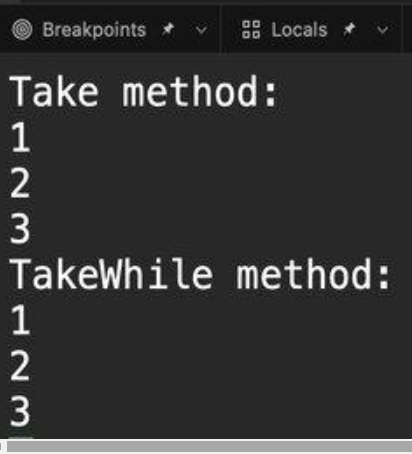• Skip: This method returns the output sequence that skips or ignores the specified number of elements from the start of the query.
• SkipWhile: This method returns the output sequence that skips the elements at the start of the query while the given predicate is matched. Skip and SkipWhile methods are often used in combination with the Take and TakeWhile methods to return a specified range of elements from a query.

Example 5:

## C#

 `using` `System; ` `using` `System.Linq; ` ` `  `public` `class` `GFG{ ` ` `  `    ``static` `public` `void` `Main (){ ` `       `  `        ``// Define a list of integers ` `        ``var` `numbers = ``new` `List<``int``>{1, 2, 3, 4, 5}; ` ` `  `        ``// Use the Skip method to skip the first 3 elements of the list ` `        ``var` `numbersAfterThree = numbers.Skip(3); ` ` `  `        ``// Output the elements of the skipped query ` `        ``foreach` `(``var` `num ``in` `numbersAfterThree) ` `        ``{ ` `            ``Console.WriteLine(num); ` `        ``} ` ` `  `        ``// Use the SkipWhile method to skip the elements of the list ` `        ``// while the elements are less than or equal to 3 ` `        ``numbersAfterThree = numbers.SkipWhile(x => x <= 3); ` ` `  `        ``// Output the elements of the skipped query ` `        ``foreach` `(``var` `num ``in` `numbersAfterThree) ` `        ``{ ` `            ``Console.WriteLine(num); ` `        ``} ` `    ``} ` `}`

Output: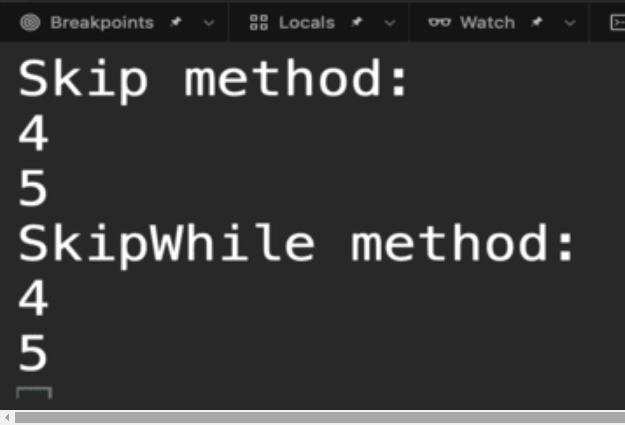## Set Operator

### Distinct:

This method is used to remove duplicate elements from a LINQ query. The Distinct method compares the elements of the query using a default equality comparer or a custom comparer that we specify, and it returns a new query that contains only the unique elements of the original query.

## C#

 `using` `System; ` `using` `System.Linq; ` ` `  `public` `class` `GFG{ ` ` `  `    ``static` `public` `void` `Main (){ ` ` `  `        ``// Define a list of integers that contains some duplicates ` `        ``var` `numbers = ``new` `List<``int``> { 1, 1, 2, 2, 3, 3, 4, 4, 5, 5 }; ` ` `  `        ``// Use the Distinct method to remove the  ` `        ``// duplicate elements from the list ` `        ``var` `uniqueNumbers = numbers.Distinct(); ` ` `  `        ``// Output the elements of the distinct query ` `        ``foreach` `(``var` `num ``in` `uniqueNumbers) ` `        ``{ ` `            ``Console.WriteLine(num); ` `        ``} ` `    ``} ` `}`

Output: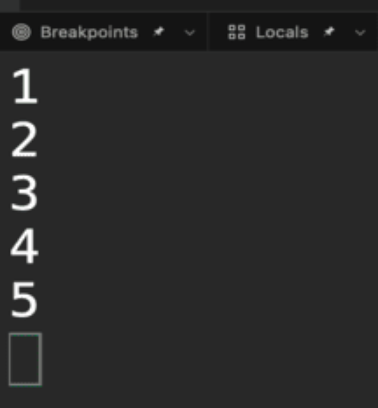In conclusion, one can benefit from using LINQ which provides a wide range of methods and capabilities that allow you to manipulate and transform the data. We can reduce the amount of code along with a better understanding of the intent and be assured of the safety of compile-time type checking.

My Personal Notes arrow_drop_up